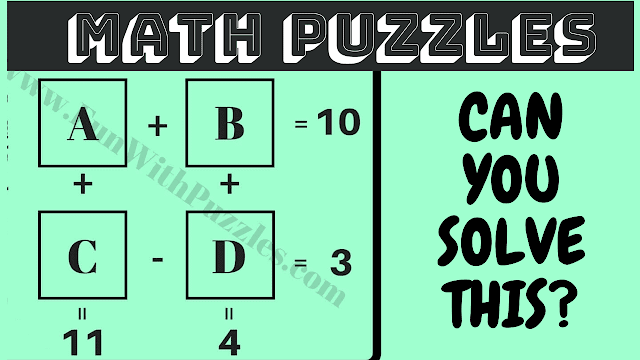Mind-Boggling Mathematical Code-Breaking Puzzle

Welcome to a brain-teasing session of mathematical code-breaking puzzles! If you love challenges that require you to decipher mathematical mysteries, you're in the right place. In this puzzle video, we present five intriguing code-breaking puzzles that will put your math skills to the test.

Each puzzle presents a unique set of number equations, and your mission is to break the mathematical codes to find the values of A, B, C, and D. Sounds exhilarating, doesn't it? But here's the twist—you have a mere 15 seconds to crack each puzzle. Feel that it's not enough time? No worries! You can pause the video to give yourself the extra time needed to conquer these mind-boggling puzzles.

We're confident that your mathematical prowess will shine as you tackle these challenges. And here's the best part: After you've cracked these puzzles, we invite you to share your answers in the comments. Challenge your fellow puzzle enthusiasts, compare solutions, and revel in the satisfaction of unraveling these intricate mathematical code-breakers.

So, are you ready to exercise your brain and take on these code-breaking puzzles? Press play, solve away, and let the mathematical mystery-solving adventure begin!